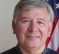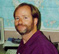## FFT complexityStarted by 6 years ago8 replieslatest reply 6 years ago885 views

In the original paper of Cooley Tukey it says that in page 298 (11) and (12) the total number of operations is T(r) = rNlogN/logr (log 2 base). if r=2 then it becomes 2NlogN

But in many books and references it says NlogN  or (1/2)*NlogN only. What is the difference here?

[ - ]The (N/2)log2(N) is a count of the number of butterfly operations on the signal flow graph. The number of complex multiplies and sum and difference operations is upper bounded by this count. For instance, the first two passes of rearrangement in time algorithm have trivial rotations of (-1)^n and (j)^n. the number of operations and data transfers is reduced by using higher radix (eg radix 4)  but at the expense of more complicated distributed scaling to accommodate bit growth.... the number of operations is further reduced by avoiding CT algorithms in favor of Good-Thomas (prime factor algorithm) with embedded Winograd algorithms for short transforms.

fred

[ - ]Some say that Cooley Tukey didn't realize that DFT is built by smaller DFT's and

in Wikipedia

https://en.wikipedia.org/wiki/Cooley%E2%80%93Tukey...

it says that

Cooley and Tukey originally assumed that the radix butterfly required O(r2

work and hence reckoned the complexity for a radix r to be O(r2N/r logrN) = O(N log2(N) r/log2r);

This analysis was erroneous, however: the radix-butterfly is also a DFT and can be performed via an FFT algorithm in O(r log r) operations, hence the radix r actually cancels in the complexity O(r log(r) N/r logrN)

In basic Butterfly there are 2 complex multiplies and 2 complex adds in simplified butterfly there are 1 complex multiply and 2 complex additions.

How did Cooley Tukey assume the complexity of butterfly as O(r2) instead of O(r log r)?

[ - ]The radix 2 DIT or DIF FFT has log_2(N) stages of N/2 butterflies each.

Each butterfly contains one complex multiply with the W_N^nk term (the twiddle factor), thus giving the famous 1/2 N log_2 N complex multiplications. Please note that this is actually an overestimate if you are doing a careful implementation, since there are many multiplications by W^0=1. In fact, there are only N/2 - 1 nontrivial multiplications per butterfly for all stages except the first in the DIT (last in the DIF), and that first one has no nontrivial multiplications at all, so a better answer is (log_2 N-1) times (N/2 - 1). There are also multiplications by W_N^(N/2) = -1, which are really subtractions and not multiplications.

Each butterfly also has 1 complex addition and 1 complex subtraction, so add these to your complexity.

Also, there is the bit reversal stage, which you might have to count (but not for DSP chips who perform this using an addressing option).

Now, to convert to real multiplications there are two options. The regular way counts 4 real multiplications and 2 additions for each complex multiply. However, if multiplies are too expensive you can get away with 3 real multiplies and 5 additions.

Y(J)S

[ - ]Hey, that last point is a neat trick.  New to me, so I looked it up.

Solve for A and B:

A + Bi = ( a + bi )( c + di )

Straightforward:

A + Bi = ( ac - bd ) + ( ad + bc )i

A = ac − bd

Karatsuba multiplication:

P1 = ac
P2 = bd
P3 = ( a + b )( c + d )

A = P1 − P2
B = P3 − P1 − P2

Thanks for bringing it up.

Ced
[ - ]I never heard the name Karatsuba, but it is one of those numeric tricks that old timers in numeric programming know about, but I have rarely seen mentioned in the literature.

I used to use it a lot in order to save a multiply, but on modern DSPs the difference in clocks between fixed point multiples and additions is not large enough to make it worthwhile.

The same thing goes for larger radixes. With the possible exception of the famous 842 algorithm when using general purpose CPUs, the speed-up is usually not worth the programming effort.

Same goes for Winograd FFTs. For architectures with large differences between multiplies and adds they are really efficient, but I stopped using them because of the large number of additions. Recently I heard that people are using them once again on graphics processors!

Y(J)S

[ - ]Just a few observations:

1) "many books and references" are likely all different and some the same.  Who is to know without the references?

2) Real or Complex?

3) Many of the treatments talk to "approximately" and "Order of" and "proportional to" so maybe a factor of 2 is of little consequence IF ONE IS KEEPING TRACK of what's being discussed.

It looks like the difference is a factor of 2 or maybe 2 factors of 2.

[ - ]